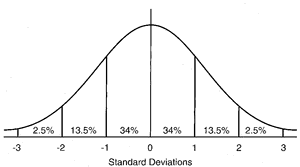# frequency distribution

(redirected from frequency distributions)
Also found in: Dictionary, Thesaurus, Financial, Encyclopedia.

## distribution

[dis″trĭ-bu´shun]
1. the specific location or arrangement of continuing or successive objects or events in space or time.
2. the extent of a ramifying structure such as an artery or nerve and its branches.
3. the geographical range of an organism or disease.
frequency distribution in statistics, a mathematical function that describes the distribution of measurements on a scale for a specific population.
normal distribution a symmetrical distribution of scores with the majority concentrated around the mean; for example, that representing a large number of independent random events. It is in the shape of a bell-shaped curve. Called also gaussian distribution. See illustration.Normal distribution. The approximate percentage of the area (or frequency) lying under the curve between standard deviations is indicated. From Dorland's, 2000.
probability distribution a mathematical function that assigns to each measurable event in a sample group the probability that the event will occur.

## fre·quen·cy dis·tri·bu·tion

a statistical description of raw data in terms of the number or frequency of items characterized by each of a series or range of values of a continuous variable.

## frequency distribution

A table or histogram showing the number of times each value of a particular variable occurs in a sample.

## frequency distribution

an arrangement of statistical data in order of the frequency of each size of the variable. For example, the numbers 2,3,5,3,4,2,1,3,4 would have the frequency distribution shown in Fig. 166. Data from a large sample often produces a NORMAL DISTRIBUTION CURVE.

## distribution

the arrangement of numerical data. The arrangement may be in accordance with magnitude, a frequency distribution, or in relation to geographical location, a spatial distribution.

age distribution
see age distribution.
bimodal distribution
the distribution has two regions of high frequency of observations separated by a zone of low frequency.
binomial distribution
a probability distribution associated with two mutually exclusive outcomes.
cluster distribution
a nonrandom distribution with observations aggregating about geographic or temporal variables. May be deceptive and merely reflect the distribution of an uneven population.
frequency distribution
a table or graph of the frequency of occurrence of each value of a variable.
Gaussian distribution
see normal distribution (below).
hypergeometric distribution
may apply to sampling without replacement of a finite population.
lognormal distribution
a distribution which is normal when the log values of the variable are considered.
normal distribution
a graph of the distribution appears as a bell-shaped curve which is symmetrical on the two sides of the vertical axis through the peak of the curve. Called also gaussian distribution.
parent distribution
the distribution (population) that was originally sampled.
Poisson distribution
regular distribution
distributed at regular intervals of time or space; all values within its given interval are equally likely.
sex distribution
an increase in frequency in one sex, which includes neutered males and neutered females. Called also sex-linked or sex-associated.
skewed distribution
a distribution in which the curve illustrating it is not symmetrical but has a long tail on one or other side of the graph.
spatial distribution
variations in distribution related to position in space, e.g. close to the door of a barn.
t-distribution
see t-test.
temporal distribution
variation in distribution related to time, e.g. occurrence of disease incidents after visits by veterinarians, inseminators, feed salesmen.

## frequency

1. the number of occurrences of a periodic process in a unit of time.
2. in statistics, the number of occurrences of a determinable entity per unit of time or of population.

cumulative frequency
the graph of its cumulative frequencies.
frequency distribution
see frequency distribution.
expected frequency
the expected number of occurrences.
observed frequency
the actual frequency; as opposed to the expected frequency.
relative frequency
the number of observations of a particular, nominated value expressed usually as a proportion of the total frequency.
total frequency
the total number of observations in the set of data.
ultrasound frequency
References in periodicals archive ?
Another way to compare the NWS and BCW phoneme frequency distributions is to estimate parameters for those distributions and to test if they are significantly different from the ones that correspond to the actual distribution of the Spanish phonemes.
3): Choice of frequency distribution for homogenous regions: After formulation of homogeneous regions, the next step was to choose the most robust frequency distribution for the homogenous regions.
Results of the CF calculations with different formulas for the consecutive frequency distribution is shown in Fig.
In this case it would seem the frequency distribution for the orbit positions cannot be built because the function has only one variable argument [v.
001) and frequency distribution of arch shapes was found by using these methods.
More data will likely reduce the confidence intervals for the frequencies of each incubation day (Figure 1), giving a clearer picture of the actual frequency distribution of all incubation periods.
Due to the fact that converting frequency distributions into unitary ones before applying the conventional expression of the Gini index is a time-consuming task, an alternative solution has been proposed.
With frequency distributions, we use interpolation formulas for the first and third quartiles quite similar to that for the median, as in equations 2.
In the light of the work presented in this article, it is anticipated that an analysis of frequency distributions of the component words of WSTs may provide indications of how better to choose which WSTs should be eliminated from a query in order to maximize retrieval effectiveness.
We have determined the frequency distributions for the analysis of DBS specimens using the rapid HPLC screening method.
More popular brands, with less skew in their purchase frequency distributions, will have less concentration, with volumes distributed more evenly across the spectrum of buyers.
The main analytical functions of the system are simple cross-tabulations and frequency distributions.

Site: Follow: Share:
Open / Close